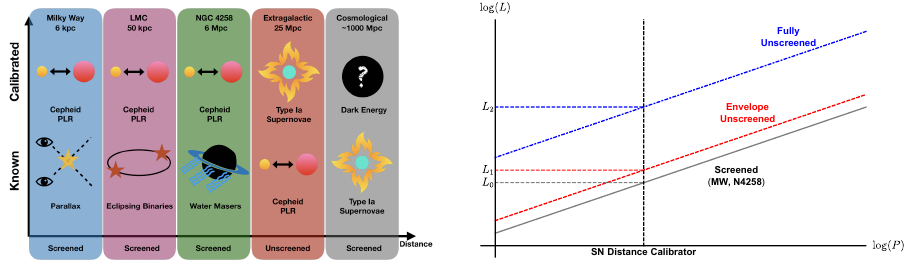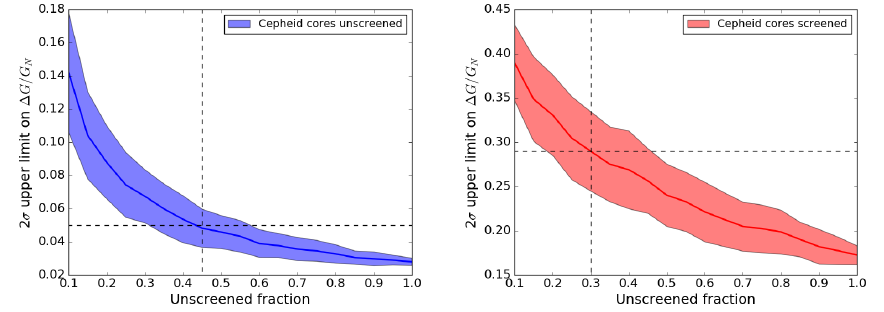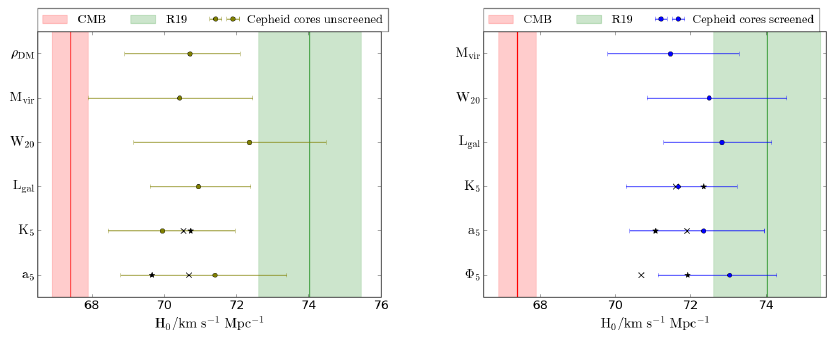A fifth-force resolution of the Hubble tension

# Background

At least on large scales, the standard cosmological model suffers from just one $$>3\sigma$$ inconsistency. This is the Hubble tension: while the local expansion rate inferred from the Cosmic Microwave Background is $$67.4 \pm 0.5$$ km s$$^{-1}$$ Mpc$$^{-1}$$, $$H_0$$ measured locally (by combining distance measurements to objects successively further away in a “cosmic distance ladder”) is $$74.03 \pm 1.42$$ km s$$^{-1}$$ Mpc$$^{-1}$$. This discrepancy is $$4.4\sigma$$, and appears to imply some form of new physics that invalidates direct comparison between low and high redshift probes of $$H_0$$ within $$\Lambda$$CDM.

A key assumption in the local measurement of $$H_0$$ is that the objects that calibrate the distance ladder – primarily Cepheid stars and Type 1a Supernovae – have identical properties between successive rungs. But in a wide variety of beyond-$$\Lambda$$CDM cosmological models which invoke so-called “screened fifth forces”, this is likely not true. Rather, while the Cepheids in the Milky Way and NGC 4258 (whose distance is measured independently by means of a water maser) will be screened by the dense environments of their hosts, those at higher redshift that calibrate supernova absolute magnitudes will be unscreened and hence feel the full fifth force. This induces a bias in the Cepheid period–luminosity relation which causes the conventional analysis to underestimate the distance to extragalactic Cepheid hosts, and hence, at fixed redshift, to overestimate $$H_0$$ (Figure 1). Thus, in such models, the expansion rate measured locally would be more in accord with that inferred from recombination.Left panel: The rungs of the cosmic distance ladder and their typical screening status. Right panel: The Cepheid period–luminosity relation when various parts of a Cepheid are unscreened. Assuming unscreened Cepheids lie on the Newtonian relation underestimates their luminosity and hence their distance.

# Unscreening the cosmic distance ladder

We have quantified these effects to flesh out this potential resolution to the Hubble tension 1. We began by formulating a set of observational proxies for the screening behaviour of Cepheids, which encompasses both well-studied screening mechanisms such as chameleon, k-mouflage and Vainshtein, a newly-proposed mechanism based on interactions between baryons and dark matter 2, and others described phenomenologically and not yet associated with an underlying theory. We then utilised the density field reconstruction of the BORG-PM model; as encapsulated in the screening maps described in an earlier post to evaluate these proxies over the Cepheids used in the distance ladder, and hence calculate the change in distance to the Cepheid hosts that the action of a screened fifth force would imply.

The magnitude of the difference depends on the strength of the fifth force. We determined maximum viable values of this in our models by means of consistency tests within the distance ladder data. The most constraining test compares the distances to galaxies measured by both the Cepheid period–luminosity relation and the tip of the red giant branch: these distances are pushed in different directions by a fifth force, so their consistency imposes a limit on the force’s strength. This is shown in Fig. 2, as a function of the fraction of galaxies that are unscreened and separately for the cases in which Cepheid cores (governing luminosity) are unscreened, or only Cepheid envelopes (governing period). This test is the strongest of its kind, and is completely agnostic as to the nature or origin of the modification to gravity.Constraints on fifth-force strength (relative to gravity) from comparing Cepheid and tip-of-the-red-giant-branch distances, as a function of the fraction of galaxies that are unscreened. Dashed lines indicate typical unscreened fractions in our models.

# $$1.5\sigma$$ consistency of local and CMB $$H_0$$

Setting the screening threshold to ensure that the galaxies that calibrate the period–luminosity relation (N4258 and the MW) are screened, and imposing the bound on fifth-force strength from Fig. 2, we calculated the maximum reduction in the inferred $$H_0$$ that each model could afford. Our results are shown in Fig. 3. While models that only unscreen Cepheid envelopes (right panel) can reduce the tension with Planck to $$\gtrsim2\sigma$$, those that unscreen cores (among them the baryon–dark matter interaction model, a dark energy model that is otherwise very little constrained) can achieve $$1.5\sigma$$ consistency. These results reveal another possible advantage to cosmologies with fifth forces, as well as demonstrating more generally that novel local resolutions of the $$H_0$$ problem are possible.Constraints on local $$H_0$$ for each of our screening models. The most successful models reach 1.5$$\sigma$$ consistency with the Planck result, well below the level at which statistical fluctuations may account for the discrepancy.

1. Harry Desmond, Bhuvnesh Jain, Jeremy Sakstein, 2019, A local resolution of the Hubble tension: The impact of screened fifth forces on the cosmic distance ladder, submitted to Phys. Rev. D., arxiv 1907.037782. J. Sakstein, H. Desmond, B. Jain, 2019, Screened Fifth Forces Mediated by Dark Matter–Baryon Interactions: Theory and Astrophysical Probes, submitted to Phys. Rev. D., arxiv 1907.03775Authored by H. Desmond
Post identifier: /method/observations/h0